Journal of Integer Sequences, Vol. 3 (2000), Article 00.2.5

## Magic Carpets

### Erich Friedman Stetson University Deland, FL 32720 Mike Keith 4100 Vitae Springs Road Salem, OR 97306 Email addresses: efriedma@stetson.edu and domnei@aol.com

Abstract: A set-theoretic structure, the magic carpet, is defined and some of its combinatorial properties explored. The magic carpet is a generalization and abstraction of labeled diagrams such as magic squares and magic graphs, in which certain configurations of points on the diagram add to the same value. Some basic definitions and theorems are presented as well as computer-generated enumerations of small non-isomorphic magic carpets of various kinds.

## Introduction

In its most general form, a magic carpet is a collection of k different subsets of a set S of positive integers, where the integers in each subset sum to the same magic constant m. In this paper we always take S = {1, 2, 3, ... n}, and refer to a magic carpet on this set as an (n, k)-carpet.

A (9,8)-carpet is shown in Figure 1, with each element of S depicted as a point (labeled with the element it represents) and each subset of S as a line connecting the points in that subset.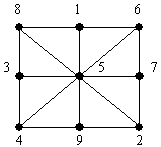Figure 1. A (9,8) magic carpet

This is just an ordinary 3x3 magic square, with each row, column, and diagonal having the same magic sum. Indeed, the motivation for this study is to generalize the notion of a magic square to an arbitrary structure on the set {1...n}, and to count and classify all non-isomorphic carpets on n points. By doing so, all possible "diagrams" of this type, in which points are labeled by {1...n} and whose lines or circles or other geometric elements pass through points with the same sum, can be generated. By omitting labels, such a diagram turns into a puzzle whose object is to determine the magic numbering. For example, the seven intersections in Figure 2 can be numbered with {1...7} such that the circle and each of the two ellipses sum to the same value. Can you verify that this is a (7,3) magic carpet by finding such a numbering? (The answer is given later, in Figure 4.)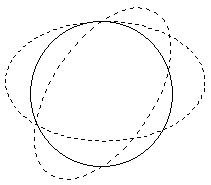Figure 2. A 7-point diagram that can be magically numbered.

A magic carpet is a generalization of other structures which have appeared in the literature, such as magic circles , magic stars , and magic graphs .

## Definitions

Denote the subsets of S by S1, ..., Sk. Let element i in S be included ("covered") ci times in the union of all the Si. The thickness of a carpet is t = min{ci} and its height is h = max{ci}. Since t <= h, there are two cases: a smooth carpet with t = h, or a bumpy one with t < h. Because of the analogy with magic squares, holey carpets with t = 0 are not very interesting, since we would like each element of S to be covered at least once (or, equivalently, for every number from 1 to n to be used in labeling the figure). In fact, a magic square has t = 2, so we are also less interested in the thin carpets with t = 1. Instead, we prefer to concentrate on plush carpets with t >= 2.

Let the subset Si have ei elements. Define the weave of a carpet to be w = min{ei}. Again motivated by magic squares, we note that loose carpets with w = 1 are not as interesting as tight ones with w >= 2. If all the ei are equal (i.e., all subsets are the same size) then the carpet is balanced.

Example: an r x r magic square, r >= 3 odd (with rows, columns, and two diagonals having the same magic sum), is a magic carpet with n = r2, k = 2r + 2, t = 2, h = 4 and w = r. It is balanced but not smooth, since t < h. Its non-smoothness is due to the diagonals being covered three times and the central square four times, while the rest are only covered twice. If the diagonals are omitted (so that we have a so-called semi-magic square) then it becomes smooth. In either case, it is plush (since t = 2) as well as tight (since w >= 2).

Define a basic magic carpet to be one that is both plush and tight. Two magic carpets are isomorphic if they are equivalent under some permutation of the elements of S. (Of course, equality of the collection of subsets is made without regard to order of the subsets.) The motivation for this definition is that two carpets which are equivalent under a permutation of S correspond to two different magic numberings of the same "figure"; i.e., we seek magic carpets with the same basic structure. In other words, we wish to enumerate all essentially different magic-numbering puzzles (blank diagrams), not all distinct solutions (labeled diagrams).

## Results

The primary combinatorial problem is to determine B(n) or B(n,k), the number of non-isomorphic basic magic carpets with given parameters. We also denote by M(n,k,t,h) the number of magic carpets (basic or not) of type (n,k,t,h).

Theorem 1: B(n) = 0 for n < 5, B(5) = 1, B(n) >= 1 for n >= 6.

Proof: The values for n <= 5 are easily obtained by direct enumeration. For n > 5, observe that any (n, k) magic carpet can be extended to an (n+1, k) carpet by taking each Si, adding 1 to each of its elements, then appending the element "1".

The unique smallest basic magic carpet, with (n,k,t,h) = (5,3,2,2), can be drawn as shown in Figure 3. It is smooth but not balanced.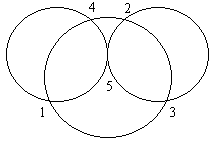Figure 3. The unique (5,3) basic magic carpet.

Theorem 2: M(n,k,t,h) = M(n,k,n-h,n-t).

Proof: From each (n,k,t,h) carpet, form another one by taking the complements of the Si.

Two carpets which are related by complementation of the subsets are called duals. Note that if C' is the dual of carpet C that has magic constant m, then C' has magic constant Tn - m, where Tn = n(n+1)/2, the nth triangular number.

We now ask a fundamental question: for a given n, which values of k admit a basic magic carpet? From the definitions it is clear that 2 <= k <= 2n - n - 1 (the latter being the number of subsets with at least two elements); however, the actual range of k is considerably smaller than this.

Theorem 3: There is a basic (n,k) magic carpet if and only if n >= 5 and 3 <= k <= q, where q is the largest coefficient in the polynomial

 n P(n) =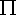(1+xi) i=1

Proof: See Theorem 1 for the proof that n >= 5 is necessary and sufficient. Obviously k cannot be 2, because two distinct subsets of {1...n} cannot cover all elements twice. Thus k >= 3 is necessary. That k <= q is necessary is trivial, since the coefficient of xj in P(n) is the number of distinct subsets of {1...n} whose elements sum to j, and q is by definition the maximum coefficient.

We now show that 3 <= k <= q is sufficient.

Let dj(n) be the coefficient of xjin the polynomial P(n) given above. Note that the sequence dj(n) is symmetric:

 dj(n) = dTn - j(n). (*)

Let

 q(n) = max dj(n) j

which equals the maximal number of subsets of {1,...n} that have the same sum. Finally, define m(n) to be the largest integer such that dm(n)(n) = q(n).

Lemma 1: Tn/2 <= m(n) <= Tn - 5.

Proof: The first inequality follows from (*). For n >= 4, d0, d1, d2, d3, d4, d5 = 1,1,1,2,2,3. Since d5(n) > dj(n) for 0 <= j <= 4, (*) gives dTn - 5(n) > dTn - j(n) for 0 <= j <= 4, which means that m(n) is at most Tn - 5.

Lemma 2: Let n >= 6 and 1 <= j <= n. There are at least two subsets of {1...n} that add to m(n) and contain j.

Proof: The number of subsets of {1...n} that add to m(n) and contain j is the coefficient of xm(n)-j in the polynomial

 n P(n, j) = (1+xj)-1(1+xi) i=1

We prove by induction on n that this coefficient is always at least 2.

By Lemma 1, it is necessary to show that the coefficients of xr in P(n,j) are at least 2 for Tn/2 <= r-j <= Tn - 5, or Tn/2 - j <= r <= Tn - 5 - j. If a given P(n,j) satisfies this we say that P(n,j) has property P.

The lemma is true for n=6 since the coefficients of P(n,j) are

```j=1: 101112222323222211101
j=2: 11012222233222221011
j=3: 1111123322233211111
j=4: 111212323323212111
j=5: 11122233233222111
j=6: 1112233333322111```

and each of these (as indicated by the boldface numbers) has property P.

Now consider two cases:

Case I: j <= 6. We have

 n P(n, j) = P(6, j)(1+xi) i=7

We know P(6, j) has property P (see table above), and multiplying by each factor i in the product is equivalent to shifting the vector of coefficients to the right by i places and adding to the original. This preserves property P.

 6(1+xi) i=1

which has coefficients 1112234445555444322111 and satisfies property P. Again, multiplying this by the remaining (1+xi) will preserve property P.

Lemma 3: dm(n-1)(n) = dm(n-1)(n-1) + dm(n-1)-n(n-1).

Proof: Since

 n n-1(1+xi) = (1+xn)(1+xi) i=1 i=1

it follows from the definition of dj(n) that dj(n) = dj(n-1) + dj-n(n-1). The lemma follows by setting j = m(n-1).

Lemma 4: For n >= 10, q(n-1) > 2n.

Proof: Consider the equation of Lemma 3. The left-hand side is no larger than q(n). The first term on the right-hand side is q(n-1). The second term is at least 2, because Lemma 1 says that m(n-1)-n >= Tn-1/2 - n, the right-hand side of which is at least 3 (if n >= 7), and dj(n) is at least 2 if j is at least 3. Therefore, q(n) >= q(n-1)+2.

Now note that the values of q(n), starting with n=5, are:

3, 5, 8, 14, 23, 40, 70, 124, 221, 397, ...

which is sequence A25591 in . Since q(10-1) = 23 > 210, the lemma is true for n=10. Using q(n) >= q(n-1)+2 and induction on n completes the proof.

We can now prove Theorem 3 by induction. First, it is true for n < 10 by direct construction by computer. We can construct (n,k) basic magic carpets for 3 <= k <= q(n-1) by taking an (n-1,k) carpet and appending n to each subset. By Lemma 4, this gives carpets for 3 <= k <= 2n.

Next, we construct an (n,k) basic magic carpet with k = 2n and magic constant m(n). For each j, 1 <= j <= n, we find two subsets which add to m(n) and contain j, which is possible by Lemma 2. We can add any number of additional subsets and still have a basic magic carpet, thus producing basic (n,k) carpets for 2n <= k <= q(n), and completing the proof.

## Numerical Results

Table 1 shows all values of B(n,k) up to n=8, determined by computer calculation. The initial values of B(n), starting with n=5, are 1, 10, 271, 36995, ... (sequence A55055).

 k=3 4 5 6 7 8 9 10 11 12 13 14 Total n=5 1 1 6 2 4 4 10 7 2 23 98 105 38 5 271 8 6 112 1300 5570 10090 9907 6240 2739 840 170 20 1 36995

Table 1. The values of B(n,k) for small indices.

Table 2 lists all the basic carpets for small values of n and k. For each carpet, the magic sum and the elements in each subset are listed (in a compact format: 1234 means {1,2,3,4}).

```(n,k)
Sum	Subsets```
```(5,3)	 10	1234 235 145
(6,3)	 14	2345 1346 1256
15	12345 2346 1356
(6,4)	 11	1235 245 236 146
12	1245 345 1236 246
14	2345 1346 1256 356
15	12345 2346 1356 456
(6,5)	  9	234 135 45 126 36
10	1234 235 145 136 46
11	1235 245 236 146 56
12	1245 345 1236 246 156
(7,3)	 19	13456 12457 12367
21	123456 23457 13467
(7,4)	 14	1256 356 1247 347
15	2346 1356 1347 1257
15	2346 456 1347 1257
15	12345 1356 1347 267
15	12345 456 1347 267
15	12345 2346 1257 267
16	12346 2356 2347 1357
16	12346 1456 2347 1357
16	12346 2356 1357 457
17	12356 2456 12347 2357
17	12356 2456 12347 1457
17	12356 2456 12347 1367
17	2456 12347 2357 1367
17	12356 2456 12347 467
17	12356 12347 2357 467
17	12356 12347 1457 467
18	12456 3456 12357 1467
18	3456 12357 2457 1467
18	12456 3456 12357 567
19	13456 12457 3457 12367
19	13456 12457 12367 1567
21	123456 23457 13467 12567
21	123456 23457 13467 3567
(8,3)	 24	123567 14568 23478
24	123567 123468 4578
25	124567 123568 123478
25	34567 123568 123478
28	1234567 234568 134578
28	1234567 134578 25678 ```

Table 2. The non-isomorphic basic magic carpets for small (n,k).

The (5,3) carpet was shown graphically in Figure 3. The (6,3), (6,4), (6,5), and (7,3) carpets are depicted in Figure 4 (in the same order they are listed in Table 2).

(6,3):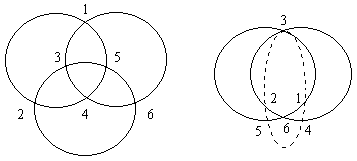(6,4)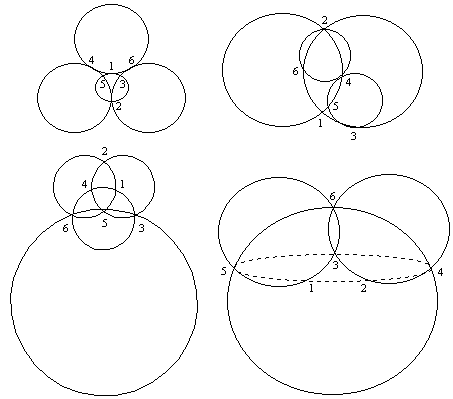(6,5)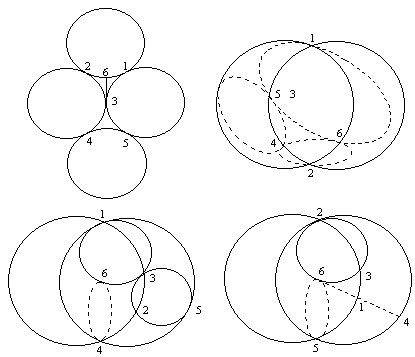(7,3)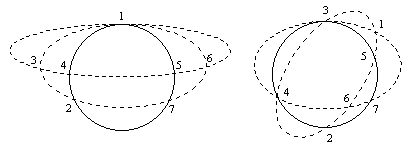Figure 4. The distinct basic magic carpets for n=6 and (n,k) = (7,3).

These figures show just one way of diagramming each magic carpet (in this case, primarily using circles and ellipses). The question of how best to visualize a carpet is primarily an aesthetic one.

The first (6,3) carpet in Figure 4 (one of the "magic circle" figures shown in ) is the smallest one that is both smooth and balanced, and also the smallest one with a high degree of symmetry (six-fold).

Since many well-known magic structures (such as magic squares and stars) are either smooth and/or balanced, it is of interest to enumerate just the smooth or balanced basic carpets. Table 3 shows the number of smooth basic carpets for all (n,k) up to n=9. The last column is sequence A55056.

 k=3 4 5 6 7 8 9 10 11 12 13 14 15 Total n=5 1 1 6 1 1 7 2 2 2 1 7 8 2 4 9 11 8 12 8 1 55 9 3 19 10 548 156 2 568 1306

Table 3. The number of smooth basic (n,k) magic carpets up to n=9.

In this table, empty cells indicate that there are no smooth carpets for that (n,k). (There are also none for n=9 and k > 15). Some of these missing (n,k) values are explained by:

Theorem 4: (n,k) basic balanced carpets can exist only if there exists a 2 <= t <= k with

tTn = 0 (mod k).

Proof: Since each element of S appears exactly t times in the union of all the subsets, the sum of all elements of the subsets is tTn. This means that the magic constant is tTn/k, which must be an integer, and so the theorem follows.

For example, for n=9 smooth carpets cannot exist, by Theorem 4, for k = 13, 14, 16, 17, 19, 22, and 23. However, this theorem does not predict all inadmissable k values - Table 3 also gives zeros for k = 4, 7, 8, 11, 18, 20, and 21. A complete characterization of which (n,k) pairs permit smooth basic carpets remains an open problem.

The largest k value which admits a smooth carpet (for n=5, 6...) is 3, 3, 8, 14, 15... (sequence A55057).

Table 4 gives the number of balanced basic carpets for all (n,k) up to n=9 (last column is sequence A55605).

 k=3 4 5 6 7 8 9 10 11 12 Total 6 1 1 7 1 1 2 1 8 1 5 12 15 4 1 38 9 2 10 73 343 699 688 367 118 22 2 2324

Table 4. The number of balanced basic (n,k) magic carpets up to n=9.

The largest k value which admits a smooth carpet (for n=6, 7,...) is 3, 5, 8, 12, 20, 32, 58, 94, 169, 289... (sequence A55606).

All balanced carpets up to n=8 are listed in Table 5.

```(n,k)
Sum	Subsets```
```(6,3)	14	2345 1346 1256
(7,3)	19	13456 12457 12367
(7,4)	15	2346 1356 1347 1257
(7,5)	12	345 246 156 237 147
16	2356 1456 2347 1357 1267
(8,3)	25	124567 123568 123478
(8,4)	18	2367 1467 2358 1458
20	13457 12467 12458 12368
21	23457 12567 13458 12468
22	23467 13567 23458 12478
27	234567 134568 124578 123678
(8,5)	16	2356 2347 1267 1348 1258
16	1456 1357 1267 1348 1258
17	2456 2357 1367 2348 1358
17	2456 1457 1367 2348 1358
18	3456 2457 1467 2358 1458
18	3456 2367 1467 2358 1458
18	3456 2457 2367 1458 1368
20	23456 13457 12467 12458 12368
21	23457 13467 12567 13458 12468
21	13467 12567 13458 12468 12378
22	23467 13567 23458 13468 12478
22	23467 13567 23458 12568 12478
(8,6)	13	346 256 247 157 238 148
16	2356 1456 2347 1357 1348 1258
16	2356 1456 2347 1267 1348 1258
16	2356 1456 1357 1267 1348 1258
16	2356 2347 1357 1267 1348 1258
16	1456 2347 1357 1267 1348 1258
17	2456 2357 1457 1367 2348 1358
17	2456 2357 1457 1367 2348 1268
17	2456 2357 1457 2348 1358 1268
18	3456 2457 2367 1467 2358 1458
18	3456 2457 2367 1467 1458 1368
18	2457 2367 1467 2358 1458 1368
18	3456 2457 2367 1458 1368 1278
21	23457 13467 12567 13458 12468 12378
22	23467 13567 23458 13468 12568 12478
(8,7)	16	2356 1456 2347 1357 1267 1348 1258
17	2456 2357 1457 1367 2348 1358 1268
18	3456 2457 2367 1467 2358 1458 1368
18	3456 2457 2367 1467 1458 1368 1278
(8,8)	18	3456 2457 2367 1467 2358 1458 1368 1278```

Table 5. All balanced basic carpets up to n=8.

Finally, Table 6 gives the number of smooth and balanced basic carpets up to n=9, and Table 7 lists them explicitly.

 k=3 4 5 6 7 8 9 Total 6 1 1 7 0 8 2 2 1 5 9 1 2 6 9

Table 6. The number of smooth-and-balanced basic (n,k) magic carpets up to n=9.

```(n,k)
Sum	Subsets```
```(6,3)	14	2345 1346 1256
(8,4)	18	2367 1467 2358 1458
27	234567 134568 124578 123678
(8,6)	18	2457 2367 1467 2358 1458 1368
18	3456 2457 2367 1458 1368 1278
(8,8)	18	3456 2457 2367 1467 2358 1458 1368 1278
(9,3)	30	234678 125679 134589
(9,6)	15	357 267 348 168 249 159
30	234678 135678 234579 125679 134589 124689
(9,9)	20	2567 3458 1568 2378 1478 2459 2369 1469 1379
20	3467 2567 3458 1568 1478 2459 2369 1379 1289
20	3467 2567 3458 1568 2378 2459 1469 1379 1289
25	24568 23578 14578 13678 23569 14569 23479 12679 13489
25	34567 24568 14578 13678 23569 23479 12679 13489 12589
25	34567 24568 23578 13678 14569 23479 12679 13489 12589 ```

Table 7. All basic basic carpets that are both balanced and smooth, up to n=9.

Note that these appear in dual pairs, unless (a) one is a self-dual, like the first (8,4) example, or (b) the dual is not a 2-cover, like the second (8,4) example.

## References

 Dudeney, H. E., "536 Puzzles and Curious Problems", Scribner's, 1967.

 Hartsfield, N. and Ringel, G. Pearls in Graph Theory: A Comprehensive Introduction, Academic Press, 1990.

 Kordemsky, B., "The Moscow Puzzles", Scribner's, 1972